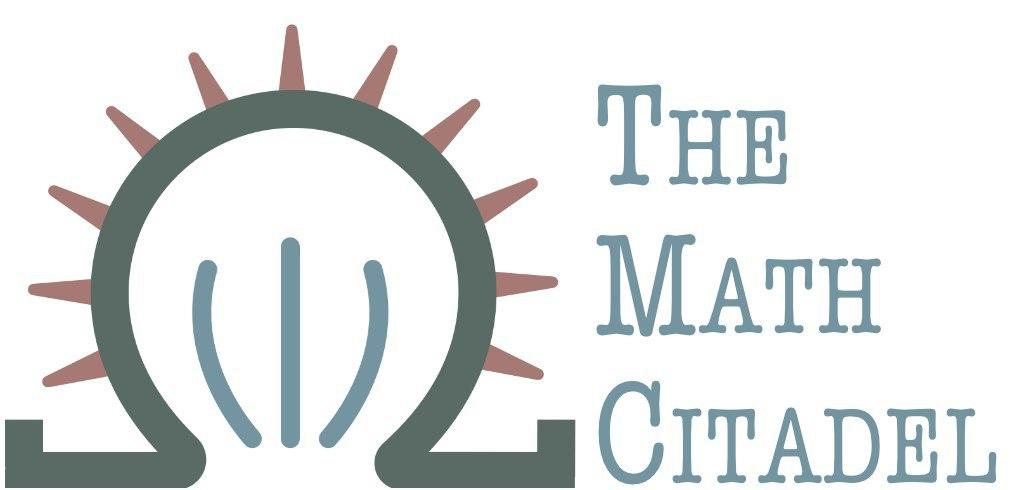# Book Reviews

## Applied and Engineering Mathematics

Includes texts that are focused on applied mathematics such as control theory, dynamical systems, queueing theory, reliability theory, signal processing, and finite element methods.

## Fundamentals

Texts that discuss the foundations of most mathematics: calculus, logic, set theory, and trigonometry

## Recreational Mathematics

Books with puzzles, problems, and general curiosities

## Algebra

Modern/abstract algebra, category theory, linear algebra, and matrix theory

## Geometry

One of the broadest topics in mathematics with many flavors: algebraic, differential, Euclidean, non-Euclidean...

## Rudimentary Mathematics

Includes mathematics at the primary and secondary levels such as arithmetic and basic algebra

## Analysis

Texts in calculus of variations, complex analysis, functional analysis, numerical analysis, real analysis, and general mathematical analysis

## Number Theory

Texts full of the study of numbers, both basic and quite advanced

## Statistics and Data Science

Works practical and theoretical in computational statistics, design of experiments, linear models, nonparametric statistics, Bayesian statistics, theoretical statistics, inference, and time series analysis

## Differential and Integral Equations

Theoretical and applied differential equations, partial differential equations, and integral equations

## Probability

Separated from statistics, this includes the study of probability from algebraic, analytic, and geometric perspectives, as well as stochastic analysis and stochastic processes.

## Topology

A branch of mathematics so fundamental it needs its own category.

## Discrete Mathematics

Includes Boolean mathematics, combinatorics, cryptography, and graph theory

## Aerospace and Mechanical Engineering

Covers many aspects of mechanical and aerospace engineering, including FEM, structural analysis, flight dynamics, etc.

## Civil Engineering

traffic engineering, roads, bridges and environmental engineering

## Materials Engineering

Books discussing materials and their properties, and their uses

the engineering side of signal processing, radio hardware, and the study of sound

## Electrical Engineering

Circuits, power generation, control, motors, electrical hardware

## Network and Industrial Engineering

Inventory management, computer networks, supply chain management, optimization

## Chemical Engineering

Fluid dynamics, water treatment, and other chemical engineering topics

## Operations Research

Optimization of manufacturing and business operations

## Systems Engineering

"Meta-engineering"